### Home > CALC > Chapter 2 > Lesson 2.4.1 > Problem2-140

2-140.
1. WHICH IS BETTER? Part Three
Below is a comparison of using a different number of rectangles to approximate the same area under a curve for f(x). Decide which will best approximate A(f, axb). Explain why.
If, in each situation, the rectangles all had equal widths, write expressions to find the area under the curve. Homework Help ✎

2.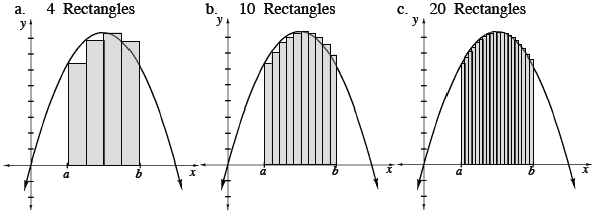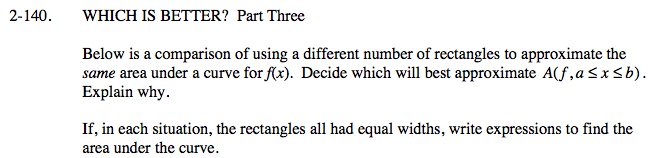Look at the shaded area beneath each curve. Which sketch most accurately represents the actual area under the curve?

General form for left-endpoint Riemann sum using sigma notation: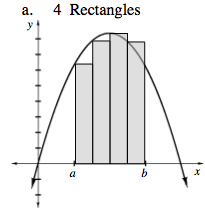$\sum_{i=0}^{3}\frac{b-a}{4}f\left ( a+\frac{b-a}{4}i \right )$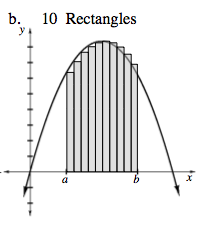$\sum_{i=0}^{9}\frac{b-a}{10}f\left ( a+\frac{b-a}{10}i \right )$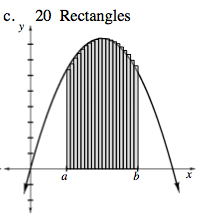$\sum_{i=0}^{19}\frac{b-a}{20}f\left ( a+\frac{b-a}{20}i \right )$